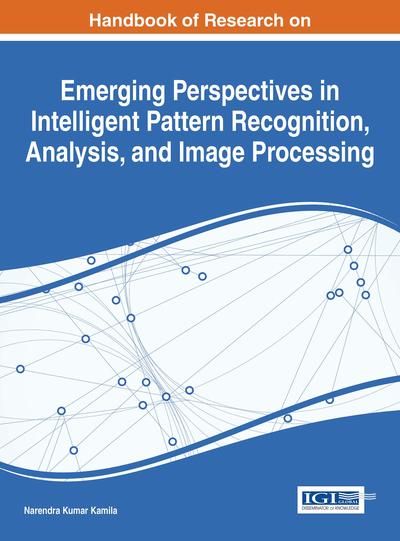# Determination of Stability of Rock Slope Using Intelligent Pattern Recognition Techniques

Swaptik Chowdhury (VIT University, India), Pratik Goyal (VIT University, India), R. Hariharan (VIT University, India) and Pijush Samui (National Institute of Technology Patna, India)
Copyright: © 2016 |Pages: 18
DOI: 10.4018/978-1-4666-8654-0.ch009
OnDemand PDF Download:
Available
\$37.50
No Current Special Offers

## Abstract

This article adopts Minimax Probability Machine (MPM) and Extreme Learning Machine (ELM) for prediction of stability status of rock slope. The proposed MPM and ELM models use unit weight (?), cohesions (cA) and (cB), angles of internal friction (?A) and ?B, angle of the line of intersection of the two joint-sets (?p), slope angle (?f), and height (H) as input parameters. For this chapter the determination of stability of rock slope has been adopted as classification problem. The developed MPM and ELM have been compared with each other. The results of this article shows that the developed MPM is robust model for prediction of stability status of rock slope.
Chapter Preview
Top

## Introduction

Slope instability is a complex phenomenon which can occur at many scales and due to many reasons. Its accurate estimation is difficult due to the complexity of physical system itself and the difficulty involved in determining the necessary input data associated with the geotechnical parameters (Sakellariou & Ferentinou, 2005). Approximately 35 landslides were recorded according to emergency events database (EM-DAT) in 2008, in which 3924 peoples died and 3828660 peoples were affected. The stability of rock slope plays very significant role in underground as well as surface works such as foundation of structures (Power houses, dams and buildings works), transportation options (canals, pipelines, roadways, & railways etc.) particularly in hilly and high terrain areas. For mining engineering works, it is essential to maintain the rock slope in stable condition during the construction time as well as in the operation time during large and small scale size underground and open excavation works. Before the advent of computationally complex methods, slope stability was usually analyzed by methods of limit equilibrium .In this method, factor of safety is derived to analyze the slope stability (Sakellariou & Ilias, 1997; Roussos, 2000).The factor of safety (FS) is the ratio of reaction over action, which is represented through moments or forces and eventually in terms of stresses depending on the geometry of assumed slip surface. But one of the major disadvantage of limit equilibrium method is that it is necessary to determine the location of critical slip surface for the analysis and the corresponding minimum value of FS by testing a considerable number of possible slip surfaces. Researchers developed various methods assuming different slip surfaces such as Taylor (1937) and Bishop (1955) assumed circular shape of slip surface but (Janbu, 1954; Sarma 1975) assumed any random shape of the slip surface. But one of the major assumptions is that the soils are isotropic and homogeneous which may not be true in practical cases (Sakellariou & Ferentinou, 2005).

Several researchers have employed soft computing models such as extreme learning machine (ELM) and Minimax probability machine classification model (MPMC) in the past effectively for various geotechnical problems. Extreme learning Machine (ELM) is a simple learning algorithm for single-hidden layer feed forward neural network (SLFN) whose learning speed is faster than the traditional feed forward network learning algorithms and it also get better generalization performance. It is generally noticed that SLFN with N hidden neuron and arbitrary input weights can learn N distinct observation with insignificant error (Tamura & Tateishi, 1997; Huang, 2003). Parameters of hidden nodes are randomly selected and weights are analytically determined in ELM. The ELN algorithm has better generalization performance, smallest training error, have smallest norm of weights and runs extremely fast and thus the advantage of EXTREME LEARNING MACHINE (Huang, 2003). The Minimax Probability Machine (Lanckriet et al, 2002) is derived from a theorem which assumes positive definite covariance matrices for each of the two classes but without making any other statistical assumptions, guarantees a lower bound on the probability of misclassification for the classification approach of linear projection to one dimension followed by comparison to a decision boundary. There are many applications in literature of MPMC (Wang et al, 2010; Yang et al, 2010; Zhou et al, 2013). The use of MPMC is advantageous as it makes minimum statistical assumptions about the distribution of underlying true classification function as accurate estimation of these functions is possible only in cases of trivial analysis. The major advantage of MPMC is that it gives a control over future classifiers.

## Key Terms in this Chapter

Rock Slope: Inclined surface of rock surface.

Prediction: Forecasting depending on a previous set of defined input.

Classification: It means to either attribute to a condition of stable or failure. A value of 1 is assigned to the stable condition of rock slope while a value of - 1 is assigned to the failure condition of rock slope.

Minimax Probability Machine: A soft computing model used for classification.

Extreme Learning Machine: A soft computing model used for classification problems depending on the input.

Slope Instability: Failure of slopes due to external as well as intrinsic factors.

## Complete Chapter List

Search this Book:
Reset